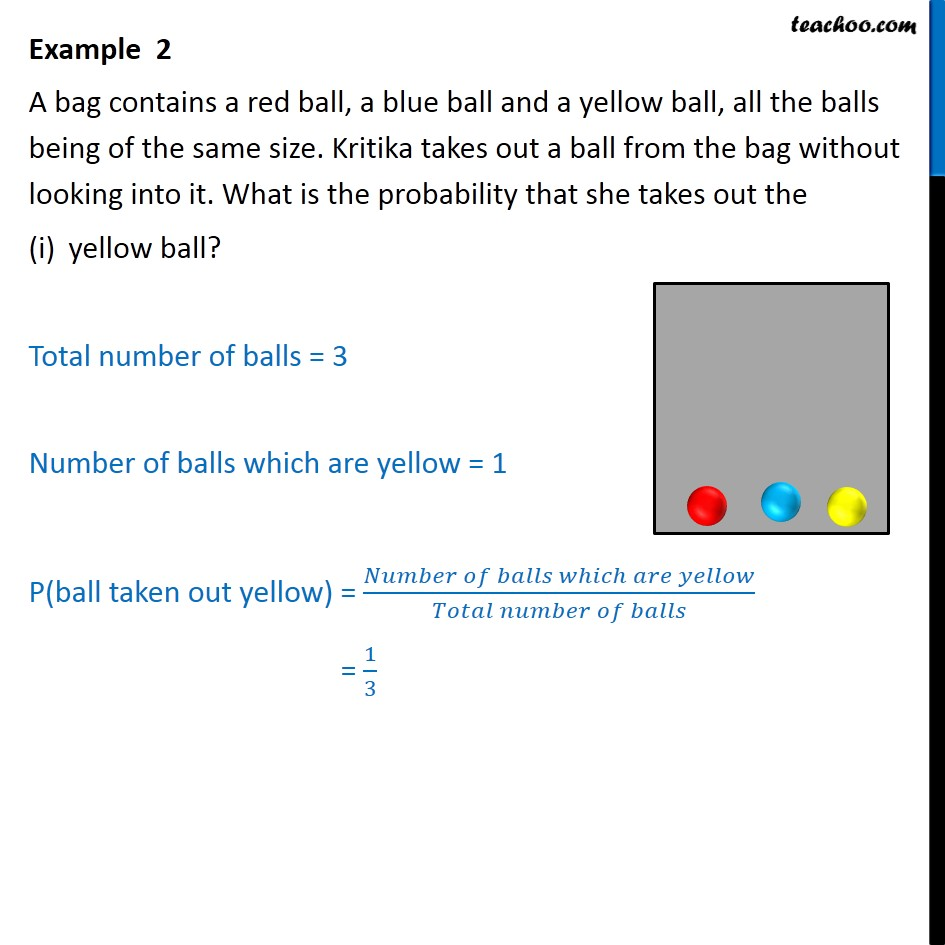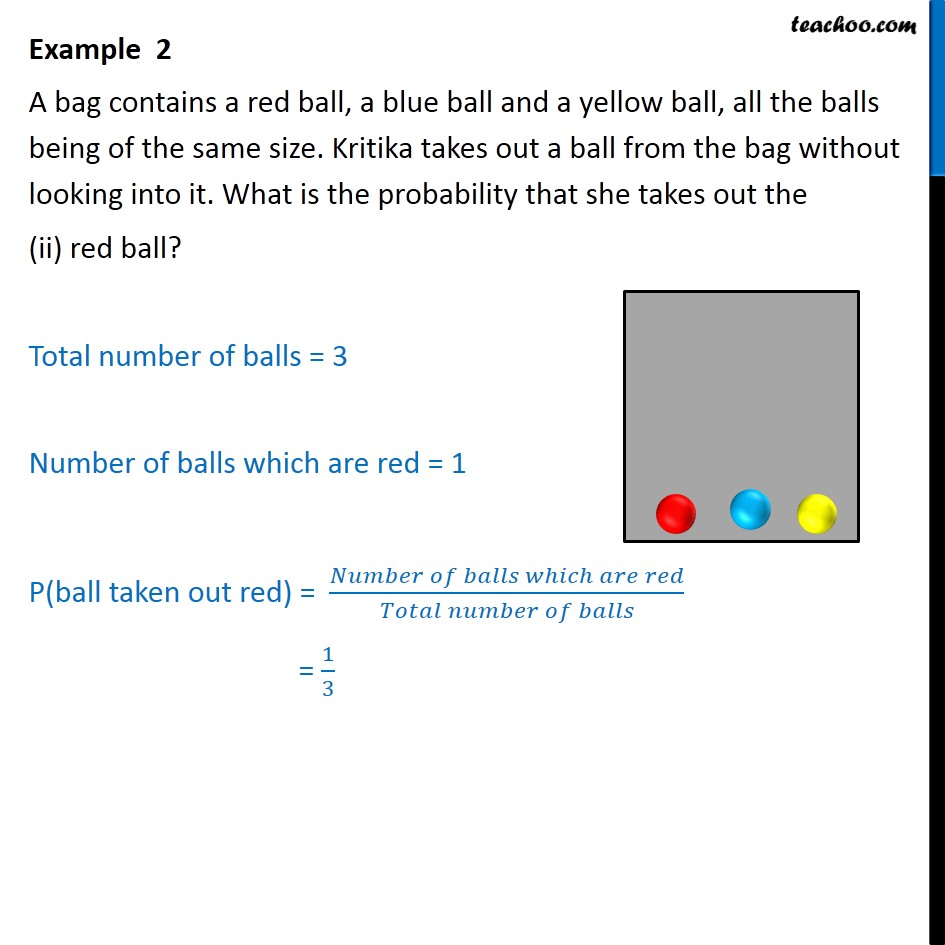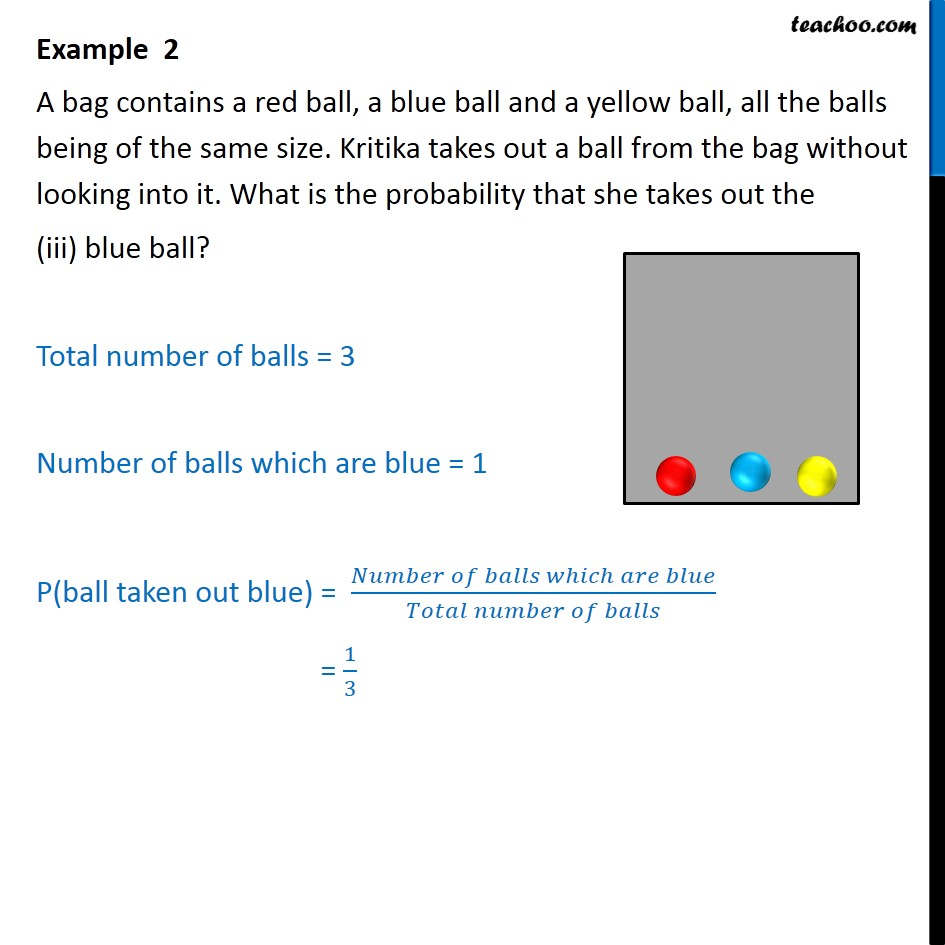1. Chapter 15 Class 10 Probability
2. Concept wise
3. Bag/ Box / Piggy bank

Transcript

Example 2 A bag contains a red ball, a blue ball and a yellow ball, all the balls being of the same size. Kritika takes out a ball from the bag without looking into it. What is the probability that she takes out the yellow ball? Total number of balls = 3 Number of balls which are yellow = 1 P(ball taken out yellow) = (𝑁𝑢𝑚𝑏𝑒𝑟 𝑜𝑓 𝑏𝑎𝑙𝑙𝑠 𝑤ℎ𝑖𝑐ℎ 𝑎𝑟𝑒 𝑦𝑒𝑙𝑙𝑜𝑤)/(𝑇𝑜𝑡𝑎𝑙 𝑛𝑢𝑚𝑏𝑒𝑟 𝑜𝑓 𝑏𝑎𝑙𝑙𝑠) = 1/3 Example 2 A bag contains a red ball, a blue ball and a yellow ball, all the balls being of the same size. Kritika takes out a ball from the bag without looking into it. What is the probability that she takes out the (ii) red ball? Total number of balls = 3 Number of balls which are red = 1 P(ball taken out red) = (𝑁𝑢𝑚𝑏𝑒𝑟 𝑜𝑓 𝑏𝑎𝑙𝑙𝑠 𝑤ℎ𝑖𝑐ℎ 𝑎𝑟𝑒 𝑟𝑒𝑑)/(𝑇𝑜𝑡𝑎𝑙 𝑛𝑢𝑚𝑏𝑒𝑟 𝑜𝑓 𝑏𝑎𝑙𝑙𝑠) = 1/3 Example 2 A bag contains a red ball, a blue ball and a yellow ball, all the balls being of the same size. Kritika takes out a ball from the bag without looking into it. What is the probability that she takes out the (iii) blue ball? Total number of balls = 3 Number of balls which are blue = 1 P(ball taken out blue) = (𝑁𝑢𝑚𝑏𝑒𝑟 𝑜𝑓 𝑏𝑎𝑙𝑙𝑠 𝑤ℎ𝑖𝑐ℎ 𝑎𝑟𝑒 𝑏𝑙𝑢𝑒)/(𝑇𝑜𝑡𝑎𝑙 𝑛𝑢𝑚𝑏𝑒𝑟 𝑜𝑓 𝑏𝑎𝑙𝑙𝑠) = 1/3

Bag/ Box / Piggy bank

About the AuthorDavneet Singh
Davneet Singh is a graduate from Indian Institute of Technology, Kanpur. He has been teaching from the past 10 years. He provides courses for Maths and Science at Teachoo.